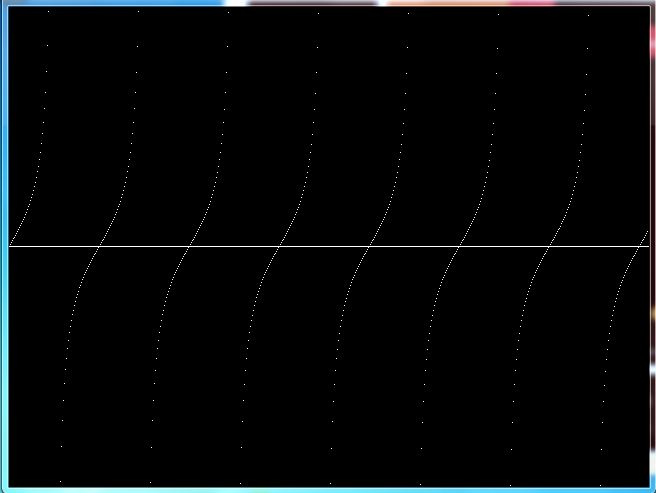# C Program to Draw Tan Graph Using C Graphics

Write a program in C to draw tan graph using graphics.h header file

In this program, we will draw tangent graph on screen. We will use putpixel functions of graphics.h header file to color a pixel at (x, y).

## C program to draw tan graph using graphics

In this program, we first initialize graphics mode, by passing graphics driver(DETECT), default graphics mode and specifies the directory path where initgraph looks for graphics drivers (*.BGI). Then we will draw a horizontal axis using line function representing the angle in radians. Inside a for loop we calculate the value of tangent for an angle and color the specific pixel using putpixel function. At last we increment the angle by 2.

```#include <conio.h>
#include <math.h>
#include <graphics.h>
#include <dos.h>

int main() {
int gd = DETECT, gm;
int angle = 0;
double x, y;

initgraph(&gd, &gm, "C:\\TC\\BGI");

line(0, getmaxy() / 2, getmaxx(), getmaxy() / 2);
/* generate a sine wave */
for(x = 0; x < getmaxx(); x++) {

/* calculate y value given x */
y = 50*tan(angle*3.141/180);
y = getmaxy()/2 - y;

/* color a pixel at the given position */
putpixel(x, y, 15);
delay(50);

/* increment angle */
angle+=2;
}

getch();

/* deallocate memory allocated for graphics screen */
closegraph();

return 0;
}
```
Program OutputRelated Topics
 C Program to draw sine wave graph on screen C Program to draw cosine(cos) wave graph C Program to draw a circle on screen C program to draw pie chart using graphics C graphics program to draw digital clock C Program to draw bar graph on screen C graphics program for moving car animation List of all C Graphics Programs